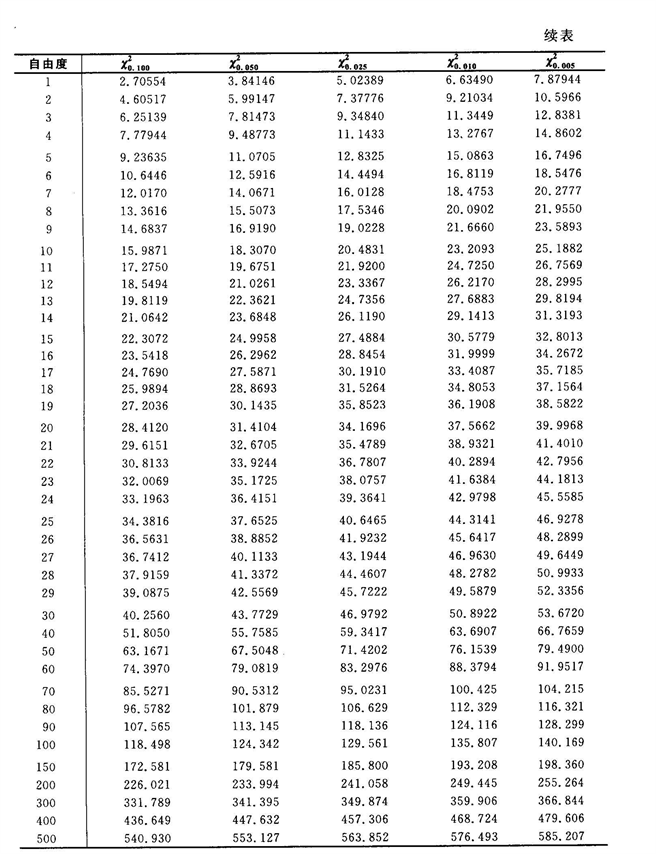﻿ Markov过程对股票价格走势的分析与预测

# Markov过程对股票价格走势的分析与预测Analysis and Forecast of Stock Price Trend Using Markov Process

Abstract: In the stock market, the stock price is a random variable which changes with time, and its chang-ing process is a random process. In this paper, the data of 104 trading days of A-share “Shanghai Pudong Development Bank (SH600,000)” from Shanghai Stock Exchange is selected. After check-ing that this process has Markov property, the corresponding Markov model is established to analyze and predict the stock price, and a relatively reasonable result is obtained. The establishment and application of the model can help us to understand the running cycle of stock price, and predict the trend of stock price.

1. 引言

2. Markov过程的基本概念

2.1. Markov过程定义

$P\left\{{X}_{n+1}=j|{X}_{0}={i}_{0},{X}_{1}={i}_{1},\cdots ,{X}_{n-1}={i}_{n-1},{X}_{n}=i\right\}=P\left\{{X}_{n+1}=j|{X}_{n}=i\right\}$

2.2. 转移概率

$P=\left({p}_{ij}\right)=\left[\begin{array}{ccccc}{p}_{00}& {p}_{0\text{1}}& {p}_{0\text{2}}& {p}_{0\text{3}}& \cdots \\ {p}_{\text{1}0}& {p}_{\text{11}}& {p}_{\text{12}}& {p}_{\text{13}}& \cdots \\ {p}_{\text{2}0}& {p}_{\text{21}}& {p}_{\text{22}}& {p}_{\text{23}}& \cdots \\ {p}_{\text{3}0}& {p}_{\text{31}}& {p}_{\text{32}}& {p}_{\text{33}}& \cdots \\ ⋮& ⋮& ⋮& ⋮& \ddots \end{array}\right]$

1) ${p}_{ij}\ge 0,i,j\in S$

2) $\underset{j\in S}{\sum }{p}_{ij}=1,\forall i\in S$

2.3. n步转移概率C-K方程

1) ${p}_{ij}^{\left(m+n\right)}=\underset{k\in S}{\sum }{p}_{ik}^{\left(m\right)}{p}_{kj}^{\left(n\right)}$

2) ${P}^{\left(n\right)}=P\cdot {P}^{\left(n-1\right)}=P\cdot P\cdot {P}^{\left(n-2\right)}=\cdots ={P}^{n}$

2.4. 随机变量的马氏性检验方法

3. 建立数学模型

3.1. 股价预测

${X}_{n}$ 是某股票在第 $n\left(n=0,1,2,\cdots \right)$ 天的收盘价格，其变化只与前一天的股票收盘价格有关， ${X}_{n}\in \left[0,+\infty \right)$。在 $\left[0,+\infty \right)$ 插入 $m-1$ 个分点 $0<{x}_{1}<{x}_{2}<\cdots <{x}_{m-1}$，分别记 ${x}_{0}$${x}_{m}$$\text{0,}\infty$，规定 ${X}_{n}\in \left[{x}_{i-1},{x}_{i}\right)$ 时出现状态i。记 ${f}_{ij}$ 为股票价格从状态i经过一个时间间隔转移到状态j的频数，记 ${p}_{ij}$ 为股票价格从状态

i经过一个时间间隔转移到状态j的概率， ${p}_{ij}={f}_{ij}/\underset{j=1}{\overset{m}{\sum }}{f}_{ij}$${p}_{ij}^{\left(n\right)}$ 表示从状态i经过n步转移到状态j的概率。

$F=\left[\begin{array}{cccc}{f}_{11}& {f}_{12}& \cdots & {f}_{1m}\\ {f}_{21}& {p}_{22}& \cdots & {f}_{m2}\\ ⋮& ⋮& \ddots & ⋮\\ {f}_{m1}& {f}_{m2}& \cdots & {f}_{mm}\end{array}\right]$

$P=\left[\begin{array}{cccc}{p}_{11}& {p}_{12}& \cdots & {p}_{1m}\\ {p}_{21}& {p}_{22}& \cdots & {p}_{m2}\\ ⋮& ⋮& \ddots & ⋮\\ {p}_{m1}& {p}_{m2}& \cdots & {p}_{mm}\end{array}\right]$

3.2. 股价的平稳分布

${\pi }_{i}$ 是系统位于状态i的平稳概率， $\pi =\left({\pi }_{1},{\pi }_{2},\cdots ,{\pi }_{m}\right)$ 为系统的平稳分布，若已知一步转移概率矩阵，则

$\left\{\begin{array}{l}\pi =\pi P\\ {\pi }_{1}+{\pi }_{2}+{\pi }_{3}+\cdots +{\pi }_{m}=1\\ {\pi }_{i}\ge 0\end{array}$

3.3. 股价的运行周期

${T}_{ij}$ 为股票价格由状态i转移到状态j所需的时间，则可推导出公式 ${T}_{ij}=1+\underset{i\ne j}{\sum }{p}_{ik}{T}_{kj}$，再通过软件求解方程组得到相应的 ${T}_{ij}$，进而求得股价的运行周期为 ${T}_{1m}+{T}_{m1}$

4. 应用实例Table 1. One-step transfer frequency table

$F=\left[\begin{array}{ccccc}7& 8& 0& 0& 0\\ 7& 22& 8& 1& 1\\ 1& 9& 19& 2& 0\\ 0& 0& 2& 10& 2\\ 0& 0& 2& 1& 2\end{array}\right]$

$P=\left[\begin{array}{ccccc}\frac{7}{15}& \frac{8}{15}& 0& 0& 0\\ \frac{7}{39}& \frac{22}{39}& \frac{8}{39}& \frac{1}{39}& \frac{1}{39}\\ \frac{1}{31}& \frac{9}{31}& \frac{19}{31}& \frac{2}{31}& 0\\ 0& 0& \frac{2}{14}& \frac{10}{14}& \frac{2}{14}\\ 0& 0& \frac{2}{5}& \frac{1}{5}& \frac{2}{5}\end{array}\right]$

${p}_{•j}=\underset{i=1}{\overset{m}{\sum }}{f}_{ij}/\underset{i=1}{\overset{m}{\sum }}\underset{j=1}{\overset{m}{\sum }}{f}_{ij}$ 可求得边际概率见表2Table 3. Calculation table of limit distribution

$\pi =\left[15/104,3/8,31/104,7/52,5/104\right]$

$\left\{\begin{array}{l}{T}_{\text{15}}=\text{1}+\frac{\text{7}}{\text{15}}{T}_{\text{15}}+\frac{\text{8}}{\text{15}}{T}_{\text{25}}\\ {T}_{\text{25}}=\text{1}+\frac{\text{7}}{\text{39}}{T}_{\text{15}}+\frac{\text{22}}{\text{39}}{T}_{\text{25}}+\frac{\text{8}}{\text{39}}{T}_{\text{35}}+\frac{\text{1}}{\text{39}}{T}_{\text{45}}\\ {T}_{\text{35}}=\text{1}+\frac{\text{1}}{\text{31}}{T}_{\text{15}}+\frac{\text{9}}{\text{31}}{T}_{\text{25}}+\frac{\text{19}}{\text{31}}{T}_{\text{35}}+\frac{\text{2}}{\text{31}}{T}_{\text{45}}\\ {T}_{\text{45}}=\text{1}+\frac{\text{2}}{\text{14}}{T}_{\text{35}}+\frac{\text{10}}{\text{14}}{T}_{\text{45}}\\ {T}_{\text{55}}=\text{1}+\frac{\text{2}}{\text{5}}{T}_{\text{35}}+\frac{\text{1}}{\text{5}}{T}_{\text{45}}\end{array}$

${T}_{\text{15}}=39.9$${T}_{\text{25}}=38.0$${T}_{\text{35}}=38.2$${T}_{\text{45}}=22.6$${T}_{\text{55}}=20.8$

${T}_{\text{11}}=6.9$${T}_{\text{21}}=11.1$${T}_{\text{31}}=14.2$${T}_{\text{41}}=19.4$${T}_{\text{51}}=17.6$

5. 结论

${\chi }^{\text{2}}$ 分布临界值表：1) 状态转移

function [z] = shaixuan(a)

for i=1:105

if a(i)>11&&a(i)<=11.24

x(i)=1;

elseif a(i)>11.24&&a(i)<=11.48

x(i)=2;

elseif a(i)>11.48&&a(i)<=11.72

x(i)=3;

elseif a(i)>11.72&&a(i)<=11.96

x(i)=4;

elseif (i)>11.96&&a(i)<=12.2

x(i)=5;

end;

end;

for i=2:105

z(i)=x(i-1)*10+x(i);

end;

2) 平稳分布

syms x1 x2 x3 x4 x5%定义符号变量，以便后续计算

p=[7/15 8/15 0 0 0;

7/39 22/39 8/39 1/39 1/39;

1/31 9/31 19/31 2/31 0;

0 0 2/14 10/14 2/14;

0 0 2/5 1/5 2/5];

eq1=x1-[x1,x2,x3,x4,x5]*p(:,1);

eq2=x2-[x1,x2,x3,x4,x5]*p(:,2);

eq3=x3-[x1,x2,x3,x4,x5]*p(:,3);

eq4=x4-[x1,x2,x3,x4,x5]*p(:,4);

eq5=x5-[x1,x2,x3,x4,x5]*p(:,5);

eq6=x1+x2+x3+x4+x5-1;

3) 求极限分布

p=[7/15 8/15 0 0 0;

7/39 22/39 8/39 1/39 1/39;

1/31 9/31 19/31 2/31 0;

0 0 2/14 10/14 2/14;

0 0 2/5 1/5 2/5];

f=[7 8 0 0 0;

7 22 8 1 1;

1 9 19 2 0;

0 0 2 10 2;

0 0 2 1 2];

p2=[15/104 39/104 31/104 14/104 5/104];

for i=1:5

for j=1:5

t(i,j)=f(i,j)*log(p(i,j)/p2(j));

end

end

4) 平均转移时间

syms x1 x2 x3 x4 x5%定义符号变量，以便后续计算

p=[7/15 8/15 0 0 0;

7/39 22/39 8/39 1/39 1/39;

1/31 9/31 19/31 2/31 0;

0 0 2/14 10/14 2/14;

0 0 2/5 1/5 2/5];

m=5;

for i=1:5

p(i,m)=0;

end;

eq1=x1-1-[x1,x2,x3,x4,x5]*p(1,:)';

eq2=x2-1-[x1,x2,x3,x4,x5]*p(2,:)';

eq3=x3-1-[x1,x2,x3,x4,x5]*p(3,:)';

eq4=x4-1-[x1,x2,x3,x4,x5]*p(4,:)';

eq5=x5-1-[x1,x2,x3,x4,x5]*p(5,:)';

NOTES

*通讯作者。

 赵婕, 赵妍. Markov链在股票市场近期走势的预测分析[J]. 现代商贸工业, 2010(16): 194-195.

 张宇山, 廖芹. 马尔可夫链在股市分析中的若干应用[J]. 华南理工大学学报, 2003(31): 74-77.

 孟银凤, 李荣华. 股票价格的马氏链预测模型[J]. 数学理论与应用, 2010(30): 53-57.

 应益荣, 吴冲锋. 股票综合指数的特征值分析法[J]. 系统工程理论方法应用, 2002(11): 177-180.

 张波，张景肖. 应用随机过程[M]. 北京: 清华大学出版社, 2004.

 张玉芬，朱雅琳. 马尔可夫性及其检验方法[J]. 价值工程, 2012, 31(2): 312-313.

Top